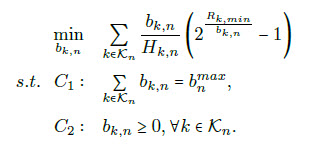# Convert problem to cvx

@Mark_L_Stone hi again, I have a new question that is related to this one. I need to add the constraint below to my cvx optimization. how can I add it to my code?

I want my cvx code to minimize the current problem that i mentioned above and it also shoud minimize the power in a way that `1/(bw.log2((1+power.gain)/bw(0.1+Interference)`) is minimized too.
this is my current cvx code:

``````cvx_begin;
variables bw(n) z(n);
minimize(sum((z-bw)./gainofthisRRH));
for i=1:n
{a(i)*log(2),bw(i),z(i)} == exponential(1);
end
sum(bw) == maxbwofthisrrh;
bw >= 0;
cvx_end;
bw = bw.';
for i=1:n
bandwidth(i)=max(0,bw(i));
end
``````

is there any way to achieve this?

You seem to have some missing symbols in your additional objective, so I am not sure what it is, and whether it is convex and expressible in CVX.

Presuming that it is convex and expressible in CVX, either combine both objectives in a way which makes sense to you., such as a weighted sum, or minimize one of the objectives subject to an additional constraint that the other objective <= u, where u is a constant you choose. If u is too small, the problem might be infeasible. By varying u, you can map out an efficient frontier of optimal tradeoffs between the two objectives.

I actually made some changes to make it easier to solve. so I came up with adding the phrase below:

``````∑k∈Kn 1/(b(w).R(k,min))
``````

to the previous one.
and b(w) is the same as the previous problem, and is the variable that I am looking for. and R(k,min) is the same R(k,min) in the previous problem, I mean currentUsersMinRate. so it’s and array in size of Kn

so I need to minimize this phrase:+ ∑k∈Kn 1/(b(w).R(k,min))

what should I add to my CVX code?

I don’t understand what problem you want to solve. Do you want to add an additional term to your existing objective function? If so, can you write this new term in MATLAB, even if is not legal in CVX? Be sure to make clear what variables would be CVX (optimization) variables and what is input data. Also, use the same variable names as before for anything which is supposed to be the same variables as before. It might be best to show the complete program. At least that way we can see the problem you want to solve.

Of course, this additional term better be convex.

Do you maybe want to use `inv_pos`? But I don’t understand what `R(k,min)` is.

yes, I want to add and additional term to my current objective function. I have calculated the second term and its is convex. so I need to minimize the sum of both phrases.
I mean something like this. (I don’t know how to add R(k,min) to my cvx.

``````cvx_begin;
variables bw(n) z(n);
minimize(sum((z-bw)./gainofthisRRH)+sum(1./bw*R(k,min));
for i=1:n
{a(i)*log(2),bw(i),z(i)} == exponential(1);
end
sum(bw) == maxbwofthisrrh;
bw >= 0;
cvx_end;
bw = bw.';``````

Can you show a formula or code segment for creating `R(k,min)` ?

R(k,min) is an array like [0.1,1,10,0.1]. it is created randomly at the beggining of the code.
its the same as currentUsersMinRate in this post. Convert problem to cvx

Does
`inv_pos(R'*bw)`
do what you want?

so it should be:

``````cvx_begin;
variables bw(n) z(n);
minimize((sum((z-bw)./gainofthisRRH)+sum(inv_pos(R'*bw)));
for i=1:n
{a(i)*log(2),bw(i),z(i)} == exponential(1);
end
sum(bw) == maxbwofthisrrh;
bw >= 0;
cvx_end;
bw = bw.';
``````

why does R has a prime? thanks

R’ because I mis-read R as a column vector.

I think you want one of the following, I’m guessing now you want the first of these:
`sum(inv_pos(R'.*bw))`
or
`inv_pos(R*bw)`

Note: edited to use R’ in first line of code

I will try both to see which one works best, thanks @Mark_L_Stone

They are different models. You have to figure out what you want.

If `R = [2 3],` they correspond to:
`1/(2*bw(1)) + 1/(3*bw(2))`
vs.
`1/(2*bw(1) + 3*bw(2))`

I need it to be like the first one. @Mark_L_Stone

I tried this one:
`minimize(sum((z-bw)./gainofthisRRH)+sum(inv_pos(MinRateOfUsers.*bw)));`

but it gives:

``````Error using  .*  (line 46)
Matrix dimensions must agree.

Error in algorithm1 (line 40)
minimize(sum((z-bw)./gainofthisRRH)+sum(inv_pos(MinRateOfUsers.*bw)));``````

Sorry, I think you need MinRateOfUsers’ . When using `.*` , the dimensions of objects before and after .* must be the same. I just edited the previous post.

thanks, but using the ’ in R, I still get the matrix dimentsion error

my CVX code is:

`````` cvx_begin;
variables bw(n) z(n);
minimize(sum((z-bw)./gainofthisRRH)+sum(inv_pos(MinRateOfUsers'.*bw)));
for i=1:n
{a(i)*log(2),bw(i),z(i)} == exponential(1);
end
sum(bw) == maxbwofthisrrh;
bw >= 0;
cvx_end;
bw = bw.';
``````

MinRateOfUsers=[0.1,1,10]
gainofthisRRH=15;
currentUsersMinRate=[0.1,1,10,0.1]
MaxBandwidthOfEachRRH=20;

You didn’t show the setting of n. If you set n = 3, the objective function will be accepted.

Also, you need to use
`currentUsersMinRate(i)`
rather than
`a(i)`
in the exponential cone constraint. However, currentUsersMinRate is 1 by 4, not 1 by 3, so you have an unused element - you should fix that in an appropriate manner.

If you get an error related to dimension, it’s a good idea to do `whos` so you can check the dimensions of all the MATLAB and CVX variables and expressions to help diagnose the error.

1 Like

thanks, I will edit my code, hope it works